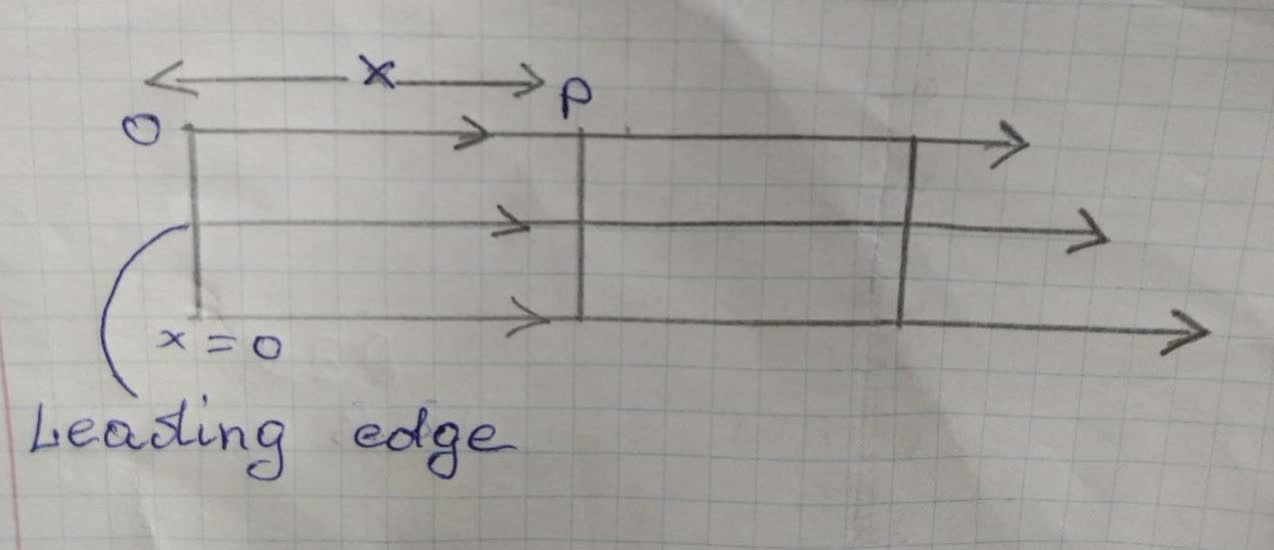# For laminar flow over a flat plate, the local heat transfer coefficienDonald Johnson 2021-12-16 Answered
For laminar flow over a flat plate, the local heat transfer coefficient ${h}_{x}$ is known to vary as ${x}^{-\frac{1}{2}}$, where x is the distance
from the leading edge (x=0) of the plate. What is the ratio of the average coefficient between the leading edge and some
location x on the plate to the local coefficient at x?
You can still ask an expert for help

• Live experts 24/7
• Questions are typically answered in as fast as 30 minutes
• Personalized clear answers

Solve your problem for the price of one coffee

• Math expert for every subject
• Pay only if we can solve itLaura Worden

We are given following data:
${h}_{x}\propto {x}^{\frac{1}{2}}$
$=K{x}^{\frac{1}{2}}$
Required:
$×\frac{\stackrel{―}{h}}{{h}^{x}}$
Using the relation (6.14) of average coefficient:
$\stackrel{―}{h}=\frac{1}{x}{\int }_{0}^{x}{h}_{x}dx$
$=\frac{1}{x}{\int }_{0}^{x}K{x}^{\frac{-1}{2}}dx$
$=\frac{1}{x}×K{\left[\begin{array}{c}\frac{{x}^{\frac{-1}{2}+1}}{\frac{-1}{2}+1}\end{array}\right]}_{0}^{x}$
$=\frac{K}{x}\left[\begin{array}{c}2x\frac{1}{2}\end{array}\right]$
$h=2K{x}^{\frac{1}{2}}$
Calculating ratio of average coefficient between the leading edge and some locaion x on the plate to the local coefficient at x
$\frac{\stackrel{―}{h}}{{h}^{x}}=\frac{2K{x}^{\frac{1}{2}}}{Kx\frac{1}{2}}$
$\frac{\stackrel{―}{h}}{{h}^{x}}=2$

We have step-by-step solutions for your answer!Barbara Meeker
Solution: We have for laminar heat flow over a flat plate the local heat transfer coefficient ${h}_{x}$,
varies as ${h}_{x},\sim C{x}^{-\frac{1}{2}}$, where ${h}_{x}$, is the coefficient at a distance x from the leading edge of the surface and the quantity C,
which depends on the fluid properties, is independent of x. Here, we
assume that ${h}_{x},=C{x}^{-\frac{1}{2}}$.
The average heat transfer coefficient between the leading edge to some location x (say at point P) is piven by$\stackrel{―}{{h}_{x}}=\frac{{\int }_{0}^{x}{h}_{x}dx}{{\int }_{0}^{x}dx}$
$=\frac{1}{x}{\int }_{0}^{x}C{x}^{-\frac{1}{2}}dx$
$=\frac{2C}{x}{\left[\begin{array}{c}{x}^{\frac{1}{2}}\end{array}\right]}_{0}^{x}=2C{x}^{-\frac{1}{2}}=2{h}_{x}$
Hence,$\frac{\stackrel{―}{{h}_{x}}}{{h}_{x}}=2$

We have step-by-step solutions for your answer!nick1337

Step1
The average heat transfer coefficient is given by as follow:-
${h}_{x}o=$ average heat transfer coefficient
${h}_{x=x}=$local heat transfer coefficient
$\stackrel{―}{{h}_{x=0}}=\frac{1}{x}{\int }_{0}^{x}{h}_{x}dx$
$\stackrel{―}{{h}_{x=0}}=\frac{1}{x}{\int }_{0}^{x}C{x}^{-1/2}dx$
$\stackrel{―}{{h}_{x=0}}=\frac{1}{x}{\int }_{0}^{x}C\frac{{x}^{-1/2+1}}{-1/2+1}dx$
$\stackrel{―}{{h}_{x=0}}=\frac{1}{x}{\int }_{0}^{x}C\frac{{x}^{1/2}}{1/2}dx$
$\stackrel{―}{{h}_{x=0}}=\frac{2}{x}\left(C{x}^{1/2}{\right)}_{0}^{x}$
Step2
after normal integration,
we get,
$\stackrel{―}{{h}_{x=0}}=2C{x}^{-1/2}$
$\stackrel{―}{{h}_{x=0}}=2×C×{h}_{x}$
hence the final result shows that the average heat transfer coefficient is twice of local heat transfer coefficient.
Therefor the ratio of average and local heat transfer coefficient = 2

We have step-by-step solutions for your answer!